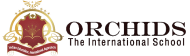Enquire Now

# Basic Calculator

A basic calculator is a free online calculator that helps you to perform different calculations in a single calculator. In today’s generation, people want to finish their work in less time To perform everyday calculations, we use Basic Calculator. A basic calculator keeps a record of values and gauges the numbers in a fraction of a second to get results. It also helps students make calculations easier, boosting their confidence in mathematics.

What is Basic Calculator?
A basic calculator consists of four functions. It can perform basic arithmetic operations such as addition, subtraction, multiplication, and division. We can easily carry it anywhere in our bags or pockets. We can also use it on mobile phones, laptops, etc.
EXAMPLES OF BASIC CALCULATOR
1. Add the following numbers 21, 43, 89, 37, 72 and 15.
To perform addition in the basic calculator. Enter all the given values using the addition operator (+), i.e.,
21 + 43 + 89 + 37 + 72 + 15 = 277
2. Find the value?
487 - 76 =?
To perform subtraction in the basic calculator. Enter the given value using the subtraction operator (-), i.e.,
487 - 76 = 411
3. Find the value?
8.2 / 7=?
To perform division in the basic calculator. Enter the given value using the division operator (/), i.e.,
8.2 / 7= 1.171
Frequently
How to Use the Basic Calculator?
1. Click on the number you want to calculate.
2. Click on the operator i.e., addition (+), subtraction (-), Multiplication (*) and division (/).
3. Click on another number you want to apply the calculation on.
4. Click on = (equals to) to get the result.
5. To reset the calculator. Click on “C”.

| K12 Techno Services ®

ORCHIDS - The International School | Terms | Privacy Policy | Cancellation